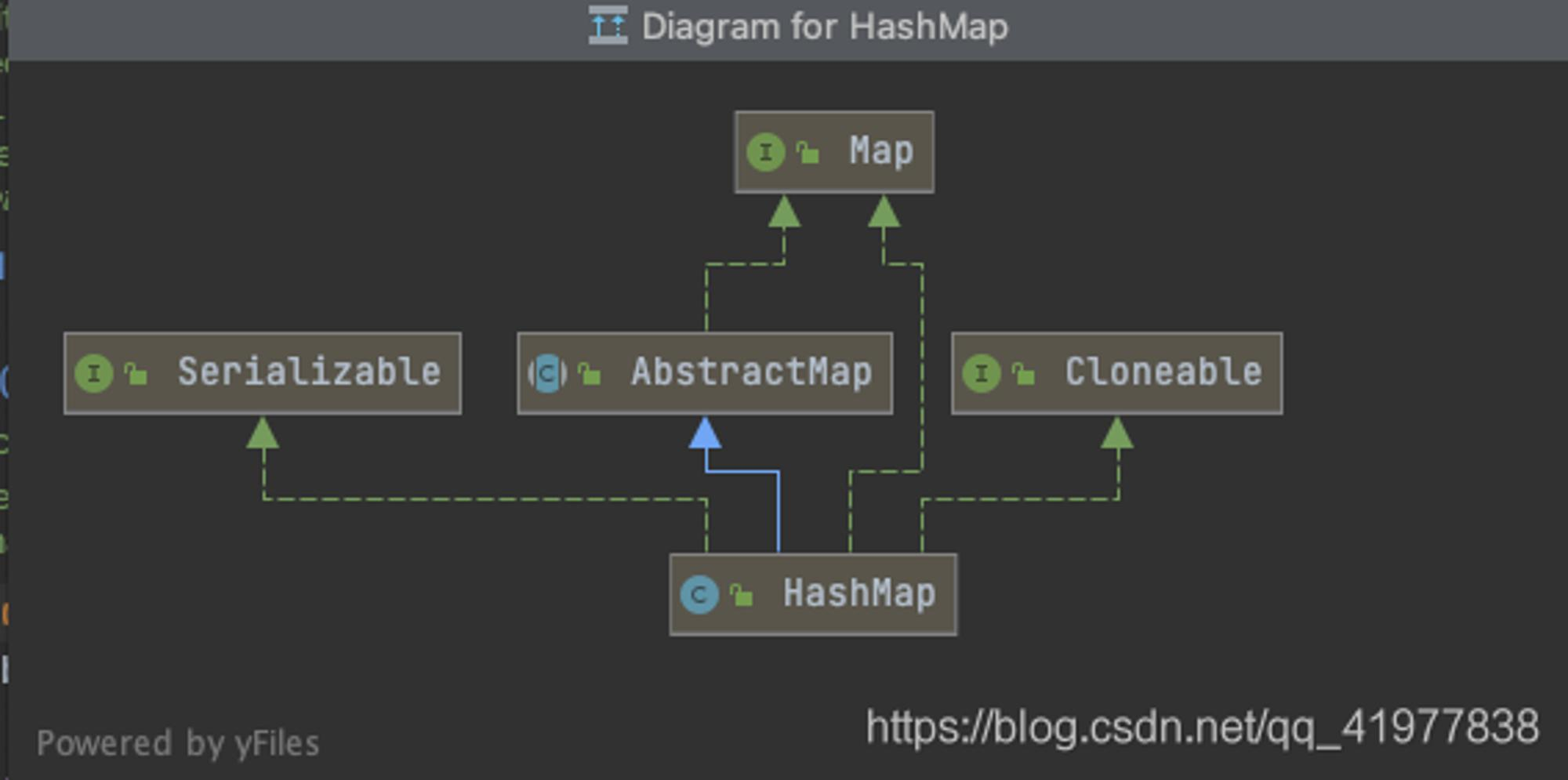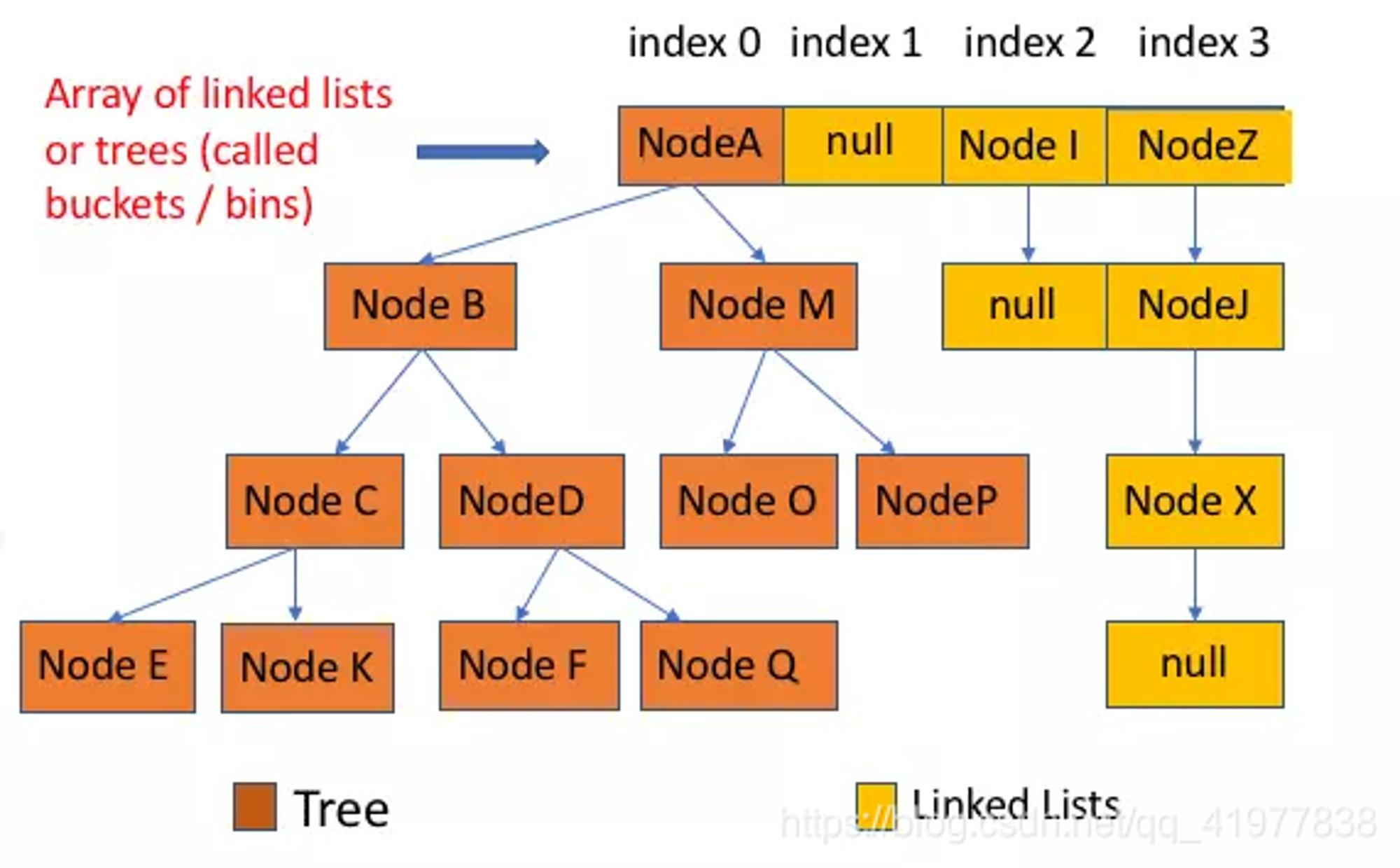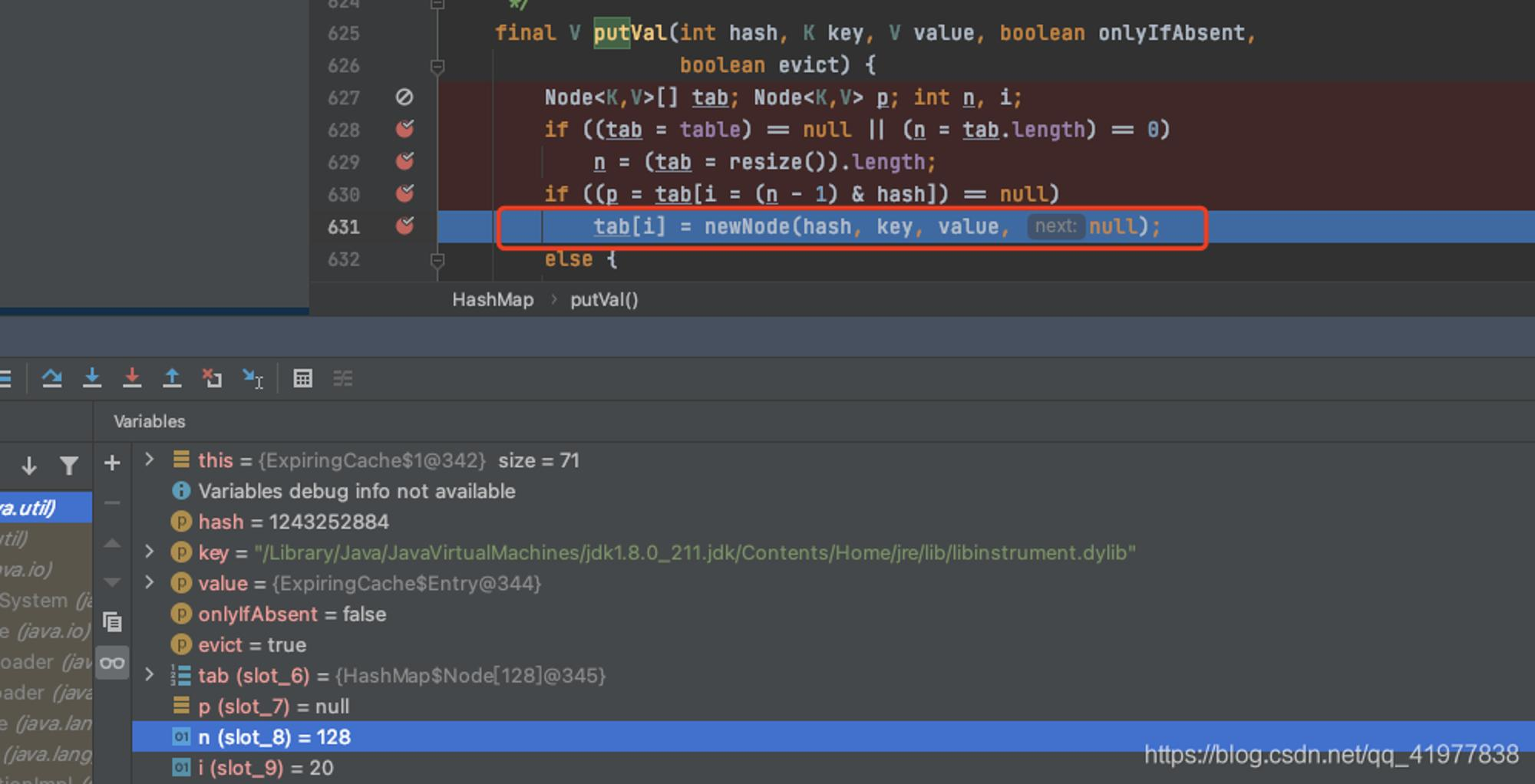墨滴ゞ浪人与酒丶

2021/04/02  阅读：80  主题：默认主题

@

简介

1. HashMap 主要用来存放键值对，它基于哈希表的 Map 接口实现，是常用的 Java 集合之一。
2. JDK1.8 之前 HashMap 由 数组+链表 组成的，数组是 HashMap 的主体，链表则是主要为了解决哈希冲突而存在的（“拉链法”解决冲突）。
3. JDK1.8 之后 HashMap 的组成多了红黑树，在满足下面两个条件之后，会执行链表转红黑树操作，以此来加快搜索速度。
4. HashMap是线程不安全的。
5. HashMap保存数据的时候通过计算key的hash值来去决定存储的位置。public class HashMap<K,Vextends AbstractMap<K,V>
implements Map<K,V>, CloneableSerializable
• 实现了 Cloneable 接口，即克隆
• AbstractMap 提供Map实现接口
• 实现java.io.Serializable接口，这意味着HashMap支持序列化，能通过序列化去传输。

内部结构

• JDK 1.8 之前 HashMap 底层是 数组和链表 结合在一起使用也就是 链表散列

• HashMap 通过 key 的 hashCode 经过 扰动函数处理过后得到 hash 值，然后通过 （n - 1）& hash 判断当前元素存放的位置 （这里的 n 指数组的长度），如果当前位置存在元素的话，就判断该元素与要存入的元素的 hash 值以及 key 是否相同，如果相同的话，直接覆盖，不相同就通过拉链法解决冲突

• 所谓的扰动函数是指 HashMap 的 hash 方法。使用 hash 方法也就是扰动函数是为了防止一些实现比较差的 hashcode() 方法 ，使用扰动函数之后可以减少碰撞

• JDK 1.8 的 hash 方法 相比于 JDK 1.7 hash 方法更加简化，但是原理不变。JDK 1.7 的 hash 方法的性能会稍差一点点，因为毕竟扰动了 4 次

• 在 HashMap 存放元素时候有这样一段代码来处理哈希值，这是 java 8 的散列值扰动函数，用于优化散列效果：

JDK 1.8 的 HashMap 的 hash 源码

static final int hash(Object key) {
int h;
// ^ ：按位异或
// >>>:无符号右移，忽略符号位，空位都以0补齐
return (key == null) ? 0 : (h = key.hashCode()) ^ (h >>> 16);
}

** JDK1.7 的 HashMap 的 hash 源码**

static int hash(int h) {
// This function ensures that hashCodes that differ only by
// constant multiples at each bit position have a bounded
// number of collisions (approximately 8 at default load factor).

h ^= (h >>> 20) ^ (h >>> 12);
return h ^ (h >>> 7) ^ (h >>> 4);
}

1. 理论上来说字符串的 hashCode是一个 int 类型值，那可以直接作为数组下标了，且不会出现碰撞。但是这个hashCode 的取值范围是[-2147483648, 2147483647]，有将近 40 亿的长度，谁也不能把数组初始化的这么大，内存也是放不下的。

2. 我们默认初始化的 Map 大小是 16 个长度 DEFAULT_INITIAL_CAPACITY = 1 << 4，所以获取的 Hash 值并不能直接作为下标使用，需要与数组长度进行取模运算得到一个下标值

3. hashMap 源码这里不只是直接获取哈希值，还进行了一次扰动计算，(h = key.hashCode()) ^ (h >>> 16)。把哈希值右移 16 位，也就正好是自己长度的一半，之后与原哈希值做异或运算，这样就混合了原哈希值中的高位和低位，增大 了随机性

Demo ：

public class test {

public static void main(String[] args) {
for (int i = 0; i < 10; i++) {
int hash = hash(new Random().nextInt());
System.out.println(hash);
}

}

static int hash(Object key) {
int h;
return (key == null) ? 0 : (h = key.hashCode()) ^ (h >>> 16);
}
}
• 使用扰动函数就是为了增加随机性，让数据元素更加均衡的散列，减少碰撞。
• 使数据分配均匀，也就是散列的效果更好，减少了 hash 的碰撞，让数据存放和获取的效率更佳。

• 在JDK1.8 之后，解决 hash 冲突就有了较大的变化

• 当链表长度阀值 （默认为8）时，会先调用 **treeifyBin()**方法。这个方法会根据 HashMap 数组来决定是否转换为红黑树。只有当数组长度大于或等于 64 情况，才会执行装欢红黑树操作，以减少搜索时间，否则就执行扩容机制public class HashMap<K,Vextends AbstractMap<K,V>
implements Map<K,V>, CloneableSerializable
{

private static final long serialVersionUID = 362498820763181265L;
/**
*  默认初始值16
*/

static final int DEFAULT_INITIAL_CAPACITY = 1 << 4// aka 16
/**
* 最大容量
*/

static final int MAXIMUM_CAPACITY = 1 << 30;
/**
* 扩容因子
*/

static final float DEFAULT_LOAD_FACTOR = 0.75f;
/**
* 当桶(bucket)上的结点数大于这个值时会转成红黑树
*/

static final int TREEIFY_THRESHOLD = 8;
/**
*  当桶(bucket)上的结点数小于这个值时树转链表
*/

static final int UNTREEIFY_THRESHOLD = 6;
/**
* // 桶中结构转化为红黑树对应的table的最小大小
*/

static final int MIN_TREEIFY_CAPACITY = 64;
/**
* 存储元素的数组
*/

transient Node<K,V>[] table;
/**
* 存放具体元素的集
*/

transient Set<Map.Entry<K,V>> entrySet;
/**
* 存放元素的个数
*/

transient int size;
/**
* 每次扩容和更改map结构的计数器
*/

transient int modCount;
/**
* 临界值 当实际大小(容量*填充因子)超过临界值时，会进行扩容
*/

int threshold;
/**
* 加载因子
*/

• 给定的默认容量为 16，负载因子为 0.75。Map 在使用过程中不断的往里面存放数据，当数量达到了 16 * 0.75 = 12 就需要将当前 16 的容量进行扩容，而扩容这个过程涉及到 rehash、复制数据等操作，所以非常消耗性能。

• 在 HashMap 中，负载因子决定了数据量多少了以后进行扩容。这里要提到上面做的 HashMap 例子，我们准备了 7 个元素，但是最后还有 3 个位置空余，2 个位置存放了 2 个元素。 所以可能即使你数据比数组容量大时也是不一定能正正好好的把数组占满的，而是在某些小标位置出现了大量的碰撞，只能在同一个位置用链表存放，那么这样就失去了 Map 数组的性能。所以，要选择一个合理的大小下进行扩容，默认值 0.75 就是说当阀值容量占了3/4 时赶紧扩容，减少 Hash 碰撞。

threshold

• threshold = capacity * loadFactor，当 Size>=threshold的时候，那么就要考虑对数组的扩增了，也就是说，这个的意思就是 衡量数组是否需要扩增的一个标准。

Node 节点类源码:

/**
* Node是单向链表，它实现了Map.Entry接口
*/

static class Node<K,Vimplements Map.Entry<K,V{
final int hash; //哈希值，存放元素到 hashMap 中用来与其他元素hash值比较
final K key;  //键
V value;   //值
Node<K,V> next;  //下一个节点

Node(int hash, K key, V value, Node<K,V> next) {
this.hash = hash;
this.key = key;
this.value = value;
this.next = next;
}

public final K getKey()        return key; }
public final V getValue()      return value; }
public final String toString() return key + "=" + value; }
//重写hashcode方法
public final int hashCode() {
return Objects.hashCode(key) ^ Objects.hashCode(value);
}

public final V setValue(V newValue) {
V oldValue = value;
value = newValue;
return oldValue;
}
//重写equals() 方法
public final boolean equals(Object o) {
if (o == this)
return true;
if (o instanceof Map.Entry) {
Map.Entry<?,?> e = (Map.Entry<?,?>)o;
if (Objects.equals(key, e.getKey()) &&
Objects.equals(value, e.getValue()))
return true;
}
return false;
}
}

Node 节点类源码:

TreeNode<K,V> parent;  // 父
TreeNode<K,V> left;    // 左
TreeNode<K,V> right;   // 右
TreeNode<K,V> prev;
boolean red;           // 判断颜色
TreeNode(int hash, K key, V val, Node<K,V> next) {
super(hash, key, val, next);
}
// 返回根节点
final TreeNode<K,V> root() {
for (TreeNode<K,V> r = this, p;;) {
if ((p = r.parent) == null)
return r;
r = p;
}

源码分析

1. 构造方法

4种构造：

//默认构造
public HashMap() {
}

//指定容量大小
public HashMap(int initialCapacity) {
}

//包含另一个“Map”
public HashMap(Map<? extends K, ? extends V> m) {
putMapEntries(m, false);
}

//指定“容量大小”和“加载因子”
public HashMap(int initialCapacity, float loadFactor) {
if (initialCapacity < 0)
throw new IllegalArgumentException("Illegal initial capacity: " +
initialCapacity);
if (initialCapacity > MAXIMUM_CAPACITY)
initialCapacity = MAXIMUM_CAPACITY;
throw new IllegalArgumentException("Illegal load factor: " +
this.threshold = tableSizeFor(initialCapacity);
}

putMapEntries 方法：

final void putMapEntries(Map<? extends K, ? extends V> m, boolean evict) {
int s = m.size();
if (s > 0) {
//判断table是否已经初始化
if (table == null) { // pre-size
// 未初始化，s 为 m 实际元素个数
float ft = ((float)s / loadFactor) + 1.0F;
int t = ((ft < (float)MAXIMUM_CAPACITY) ?
(int)ft : MAXIMUM_CAPACITY);
// 如果t大于阀值 则初始化
if (t > threshold)
threshold = tableSizeFor(t);
}
//table 已经初始化，并且m元素个数大于阀值，进行扩容处理
else if (s > threshold)
resize();
// 将所有元素添加到HashMap中
for (Map.Entry<? extends K, ? extends V> e : m.entrySet()) {
K key = e.getKey();
V value = e.getValue();
putVal(hash(key), key, value, false, evict);
}
}
}

2. put方法

HashMap 只提供了 put 用于添加元素，putVal方法 只是给put方法调用到一个方法，并没有提供给用户使用

public V put(K key, V value) {
return putVal(hash(key), key, value, falsetrue);
}

putVal 方法添加元素分析：

1. 如果定位到数组位置没有元素就直接插入
2. 如果定位到的数组位置有元素就要和插入的 key 比较 ，如果 key 相同就直接覆盖，如果key不同 ，就判断 p 是否是一个树节点，如果是就调用 e = ((TreeNode<K,V>)p).putTreeVal(this, tab, hash, key, value)将元素添加进入 ，如果不是就遍历链表插入 （插入链表尾部）
final V putVal(int hash, K key, V value, boolean onlyIfAbsent,
boolean evict)

{
Node<K,V>[] tab; Node<K,V> p; int n, i;
// table未初始化或者长度0 就进行扩容
if ((tab = table) == null || (n = tab.length) == 0)
//扩容操作
n = (tab = resize()).length;
//根据哈希值计算下标，确定元素存放到那个桶，桶为空，新生成节点放到桶中 （这个节点是放在数组中），
if ((p = tab[i = (n - 1) & hash]) == null)
tab[i] = newNode(hash, key, value, null);
//否则同种存在元素
else {
Node<K,V> e; K k;
//比较桶中第一个元素 的hash 是否相等，也是key相等 ，key存在 直接覆盖value
if (p.hash == hash &&
((k = p.key) == key || (key != null && key.equals(k))))
//将元素赋值给 e 用e来标识
e = p;
//判断该列是否为红黑树 hash值不相等，即key不想等；为红黑树结点
else if (p instanceof TreeNode)
//放入树中
e = ((TreeNode<K,V>)p).putTreeVal(this, tab, hash, key, value);
//为链表结点
else {
//在链表最末插入
for (int binCount = 0; ; ++binCount) {
//到达链表尾部
if ((e = p.next) == null) {
//插入新结点
p.next = newNode(hash, key, value, null);
//结点数量达到阀值 8 执行treeifyBin方法。这个方法根据 Hashmap 数组决定是否装换为红黑树，只有当数组大于等于 64 情况下才会转换成红黑树，以减少时间搜索，否则就是对数组
if (binCount >= TREEIFY_THRESHOLD - 1// -1 for 1st
treeifyBin(tab, hash);
//跳出循环
break;
}
//判断链表中结点的key值与插入的元素的key值是否相等
if (e.hash == hash &&
((k = e.key) == key || (key != null && key.equals(k))))
// 相等，跳出循环
break;
p = e;
}
}
// 表示在桶中找到key值、hash值与插入元素相等的结点
if (e != null) { // existing mapping for key
//记录e的value
V oldValue = e.value;
if (!onlyIfAbsent || oldValue == null)
//新值替换旧值
e.value = value;
//访问后回调
afterNodeAccess(e);
return oldValue;
}
}
++modCount;
//超过最大容量就扩容
if (++size > threshold)
resize();
afterNodeInsertion(evict);
return null;
}

1. 当调用 put 方法的时候，使用扰动函数 (h = k.hashCode()) ^ (h >>> 16) 得到最后hash值，

2. 根据 key.hash 计算 桶数组的索引 tab[i = (n - 1) & hash]) 得到key的位置

2.1 如果该位置没有数据，用该数据新生成一个节点保存新数据，返回null2.2 如果该位置有数据是红黑树，那么执行相应的 插入/ 更新操作

2.3 如果该位置有数据是链表,循环,如果链表下一个元素为null ,采用尾插法新增节点保存新数据 ,如果大于等于 8 就进行扩容（ treeifyBin(tab, hash); 方法决定 扩容数组 还是转成红黑树），判断链表中 key 与插入元素key 是 否相等，如果该链表已经有这个节点了，那么找到该节点并更新新数据，返回老数据。

• 头插法:就是说新来的值会取代原有的值，原有的值就顺推到链表中去
• 多线程中会出现死循环的情况

hashmap的扩容机制

• 创建一个新的Node空数组，长度是原数组的2倍。
• 遍历原Node数组，把所有的Node重新Hash到新数组。

Node<K,V>[] tab; Node<K,V> p; int n, i;
if ((tab = table) == null || (n = tab.length) == 0)
n = (tab = resize()).length;
if ((p = tab[i = (n - 1) & hash]) == null)
tab[i] = newNode(hash, key, value, null);

• 使用头插会改变链表的上的顺序，但是如果使用尾插，在扩容时会保持链表元素原本的顺序，就不会出现链表成环的问题了

3. get方法

public V get(Object key) {
Node<K,V> e;
return (e = getNode(hash(key), key)) == null ? null : e.value;
}
final Node<K,V> getNode(int hash, Object key) {
Node<K,V>[] tab; Node<K,V> first, e; int n; K k;
//判断是否为null
if ((tab = table) != null && (n = tab.length) > 0 &&
(first = tab[(n - 1) & hash]) != null) {
// 桶中第一个 （数组元素）相等 直接返回第一个
if (first.hash == hash && // always check first node
((k = first.key) == key || (key != null && key.equals(k))))
return first;
//// 桶中不止一个结点
if ((e = first.next) != null) {
//为红黑树结点
if (first instanceof TreeNode)
//在红黑树中查找
return ((TreeNode<K,V>)first).getTreeNode(hash, key);
//否则在链表中查找
do {
if (e.hash == hash &&
((k = e.key) == key || (key != null && key.equals(k))))
return e;
} while ((e = e.next) != null);
}
}
return null;
}

4. resize方法

• 进行扩容，会伴随着一次重新 hash 分配，并且会遍历 hash 表中所有的元素，是非常耗时的。在编写程序中，要尽量避免 resize。
final Node<K,V>[] resize() {
Node<K,V>[] oldTab = table;
int oldCap = (oldTab == null) ? 0 : oldTab.length;
int oldThr = threshold;
int newCap, newThr = 0;
// 超过最大值就不再扩容，随意碰撞吧
if (oldCap > 0) {
if (oldCap >= MAXIMUM_CAPACITY) {
// 原数组长度大于最大容量(1073741824) 则将threshold设为Integer.MAX_VALUE=2147483647
threshold = Integer.MAX_VALUE;
return oldTab;
}
//没有超过最大值，就扩容为原来的2倍
else if ((newCap = oldCap << 1) < MAXIMUM_CAPACITY &&
oldCap >= DEFAULT_INITIAL_CAPACITY)
newThr = oldThr << 1// double threshold
}
else if (oldThr > 0// initial capacity was placed in threshold
newCap = oldThr;
else {               // zero initial threshold signifies using defaults
// 默认初始化
newCap = DEFAULT_INITIAL_CAPACITY;
}
// 计算新的resize上限
if (newThr == 0) {
// 如果新 的容量 ==0
float ft = (float)newCap * loadFactor;
newThr = (newCap < MAXIMUM_CAPACITY && ft < (float)MAXIMUM_CAPACITY ?
(int)ft : Integer.MAX_VALUE);
}
// 将临界值设置为新临界值
threshold = newThr;
@SuppressWarnings({"rawtypes","unchecked"})
// 扩容
Node<K,V>[] newTab = (Node<K,V>[])new Node[newCap];
table = newTab;
// 如果原来的table有数据，则将数据复制到新的table中
if (oldTab != null) {
// 把每个bucket都移动到新的buckets中
for (int j = 0; j < oldCap; ++j) {
Node<K,V> e;
// 获取数组的第j个元素
if ((e = oldTab[j]) != null) {
oldTab[j] = null//将旧的hash桶数组在j结点处设置为空，方便gc
// 如果链表只有一个，则进行直接赋值
if (e.next == null)
newTab[e.hash & (newCap - 1)] = e;
//是否是红黑树扩容
else if (e instanceof TreeNode)
//如果是红黑树节点 split 方法扩容
((TreeNode<K,V>)e).split(this, newTab, j, oldCap);
else { // preserve order
// 链表优化重hash的代码块
Node<K,V> loHead = null, loTail = null;
Node<K,V> hiHead = null, hiTail = null;
Node<K,V> next;
do {
next = e.next;
if ((e.hash & oldCap) == 0) {
if (loTail == null)
else
loTail.next = e;
loTail = e;
}
else {
if (hiTail == null)
else
hiTail.next = e;
hiTail = e;
}
} while ((e = next) != null);
if (loTail != null) {
loTail.next = null;
}
if (hiTail != null) {
hiTail.next = null;
}
}
}
}
}
return newTab;
}

//红黑树转回链表的阈值
static final int UNTREEIFY_THRESHOLD = 6;

/** 这个方法在HashMap进行扩容时会调用到:  ((TreeNode<K,V>)e).split(this, newTab, j, oldCap);
* @param map 代表要扩容的HashMap
* @param tab 代表新创建的数组，用来存放旧数组迁移的数据
* @param index 代表旧数组的索引
* @param bit 代表旧数组的长度，需要配合使用来做按位与运算
*/

final void split(HashMap<K,V> map, Node<K,V>[] tab, int index, int bit) {
//做个赋值，因为这里是((TreeNode<K,V>)e)这个对象调用split()方法，所以this就是指(TreeNode<K,V>)e对象，所以才能类型对应赋值
TreeNode<K,V> b = this;
//设置低位首节点和低位尾节点
TreeNode<K,V> loHead = null, loTail = null;
//设置高位首节点和高位尾节点
TreeNode<K,V> hiHead = null, hiTail = null;
//定义两个变量lc和hc，初始值为0，后面比较要用，他们的大小决定了红黑树是否要转回链表
int lc = 0, hc = 0;
//这个for循环就是对从e节点开始对整个红黑树做遍历，如果对这循环赋值略有不懂，可以参考这篇模仿的博客@1
for (TreeNode<K,V> e = b, next; e != null; e = next) {
//取e的下一节点赋值给next遍历
next = (TreeNode<K,V>)e.next;
//取好e的下一节点后，把它赋值为空，方便GC回收
e.next = null;
//以下的操作就是做个按位与运算，按照结果拉出两条链表，具体的操作可以参考这篇博客@2
if ((e.hash & bit) == 0) {
if ((e.prev = loTail) == null)
else
loTail.next = e;
loTail = e;
//做个计数，看下拉出低位链表下会有几个元素
++lc;
}
else {
if ((e.prev = hiTail) == null)
else
hiTail.next = e;
hiTail = e;
//做个计数，看下拉出高位链表下会有几个元素
++hc;
}
}

//如果低位链表首节点不为null，说明有这个链表存在
//如果链表下的元素小于等于6
if (lc <= UNTREEIFY_THRESHOLD)
//那就从红黑树转链表了，低位链表，迁移到新数组中下标不变，还是等于原数组到下标
else {
//低位链表，迁移到新数组中下标不变，还是等于原数组到下标，把低位链表整个拉到这个下标下，做个赋值
//如果高位首节点不为空，说明原来的红黑树已经被拆分成两个链表了
//那么就需要构建新的红黑树了
}
}
//如果高位链表首节点不为null，说明有这个链表存在
//如果链表下的元素小于等于6
if (hc <= UNTREEIFY_THRESHOLD)
//那就从红黑树转链表了，高位链表，迁移到新数组中的下标=【旧数组+旧数组长度】
else {
//高位链表，迁移到新数组中的下标=【旧数组+旧数组长度】，把高位链表整个拉到这个新下标下，做赋值
如果低位首节点不为空，说明原来的红黑树已经被拆分成两个链表了
//那么就需要构建新的红黑树了
}
}
}ゞ浪人与酒丶

2021/04/02  阅读：80  主题：默认主题